## Crossover and Mutation

To understand the importance of the mutation operator interacting with the crossover operator one has to consider the contributions of each of these operators more concretely. The implementation of the crossover operator used in the algorithm is given below.

//***************************************************
// Apply a crossover operation onto two adjacent strings at intersection x
// The strings are assumed to be randomly paired in the area at position 1
//
// p := number of parameters between strings left and right (actually  p=5)
// l := length of strings ( actually l=5)
// n := number of elements in POP (actually n=4)

function[POP]= crossover(POP,l,p,n)

// Take a first row
r=1
while(r < n)

// printf('crossover r = %d\n',j)

// Select randomly a point in this row

x = round(l * rand()), if(x == 0) then x=1,end,

// printf('crossover x = %d\n',x)

for i=x:l, m=POP(r+1,i)
POP(r+1,i) = POP(r,i)
POP(r,i) = m
end

r=r+2
end

endfunction


According to this code the crossover operator takes two stringsembedded in the population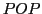in a row indicated by the index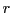in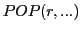and reaching from column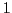to columnrepresented by the index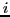.

Within a first stringa random pointis selected as point of reference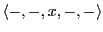.

Then will all values of the stringsfrom the selected pointuntil the end of the both strings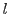be exchanged. This is shown below: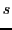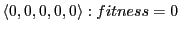(4.9)(4.10)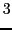(4.11)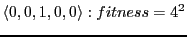(4.12)(4.13)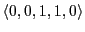(4.14)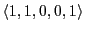(4.15)(4.16)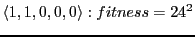(4.17)

In this example the overall number of zeros has not changed, not even there positions. If we would repeat the crossover-operation with differentvalues this would not change. The only difference would be the computed fitness value which depends from the position of the 'ones' in the string.

Using selection we could select the string with the highest fitness value twice and repeat the application of the crossover operator:(4.18)(4.19)

As one can see directly the application of the crossover operator will not change the fitness of these strings. Because they are equal in shape no exchange can change this structure furthermore.

From this follows that the application of the crossover operator only makes sense as long as the involved strings are at least in one position different.

From this one can deduce the following hypothesis when to apply the mutation operator additionally to the crossover operator.

Hypothesis: If a populationhas reached a 'sufficient' similarity between the information strings and the sum of all fitness values of these strings is 'sufficiently' below a known theoretical maximum, then one can apply mutation. This is nicely illustrated in the following examples.

The initial population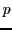has 11 zeros and a population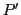generated only by crossover after 50 cycles still 8 zeros. The 'leading' zeros have been selected out by the fitness values.

  (P) POP  =

0.    1.    1.    0.    1.    13.    169.    0.    0.    0.
1.    1.    0.    0.    0.    24.    576.    0.    0.    0.
0.    1.    0.    0.    0.    8.     64.     0.    0.    0.
1.    0.    0.    1.    1.    19.    361.    0.    0.    0.


 (P') POP  =

column 1 to 9

1.    1.    0.    0.    1.    28.    784.    0.2774239    1.1096957
1.    1.    0.    0.    0.    25.    625.    0.2211607    0.8846426
1.    1.    1.    0.    0.    24.    576.    0.2038217    0.8152866
1.    1.    1.    0.    1.    29.    841.    0.2975938    1.1903751The population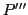is generated during a run of 80 cycles using crossover and every 10th cycle mutation. This population contains only 4 zeros and this population can be improved further.

 (P''') POP  =
column 1 to 9

1.    1.    1.    0.    0.    31.    961.    0.3085072    1.2340289
1.    1.    1.    1.    1.    23.    529.    0.1698234    0.6792937
1.    1.    1.    1.    1.    29.    841.    0.2699839    1.0799358
1.    0.    1.    0.    1.    28.    784.    0.2516854    1.0067416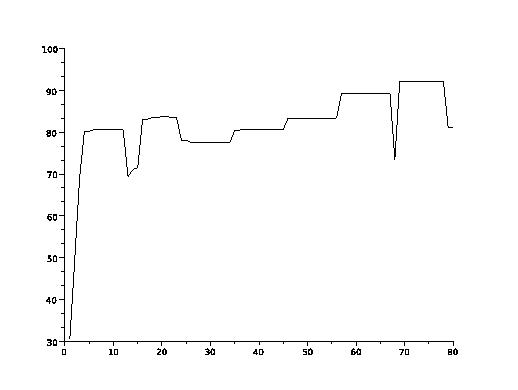Gerd Doeben-Henisch 2013-01-14